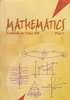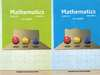Share

Books Shortlist

# Textbook Solutions for CBSE (Arts) Class 12 - CBSE

< prev 1 to 2 of 2 next >NCERT Solutions for Class 12 Mathematics Textbook Mathematics

Chapters : Relations and Functions, Inverse Trigonometric Functions, Matrices, Determinants, Continuity and Differentiability, Application of Derivatives, Integrals, Application of Integrals, Differential Equations, Vector Algebra, Three Dimensional Geometry, Linear Programming, ProbabilityRD Sharma Solutions for Mathematics for Class 12 by R D Sharma Mathematics

Chapters : Relations, Functions, Binary Operations, Inverse Trigonometric Functions, Algebra of Matrices, Determinants, Adjoint and Inverse of a Matrix, Solution of Simultaneous Linear Equations, Continuity, Differentiability, Differentiation, Higher Order Derivatives, Derivative as a Rate Measurer, Differentials, Errors and Approximations, Mean Value Theorems, Tangents and Normals, Increasing and Decreasing Functions, Maxima and Minima, Indefinite Integrals, Definite Integrals, Areas of Bounded Regions, Differential Equations, Algebra of Vectors, Scalar Or Dot Product, Vector or Cross Product, Scalar Triple Product, Direction Cosines and Direction Ratios, Straight Line in Space, The Plane, Linear programming, Probability, Mean and Variance of a Random Variable, Binomial Distribution

< prev 1 to 2 of 2 next >

## Textbook solutions and Reference book solutions for Class 12

• On this page you will find NCERT solution, RD Sharma solution for Class 12.
• Each chapter comes with subsections that provide in-depth knowledge about the basic concepts.
• Solved equations along with examples help the students to understand the different steps towards solving their exercises.
• Compilation of different exercises makes it a great choice for students to practice to brush up their concepts and skills.
• These books are a must-have for the students who want to closely evaluate the different aspects of their respective subjects.
• Different graphs along with schematic representations help the students to memorise the many steps.
• These solutions for Class 12 are prepared by experts, include the problems from latest edition and are as per CBSE pattern.

## Reference book solutions and textbook solutions on Shaalaa.com

Shaalaa.com is a great source for Reference book solutions and textbook solutions of all Subjects for Practice. Our best teachers have reviewed all the Class 12 solutions of exercise questions that will help you solve all the Class 12 questions of all subject without any problem. Reference book Solutions are highly beneficial especially when you are thinking to prepare for school as well as competitive level examinations. We at Shaalaa.com work hard to create better ways in order to help students and provide you with the detailed solutions for all questions from reference boook and textbook as per the latest marking scheme pattern is to back you up in effective learning in all the examinations conducted by CBSE.
S